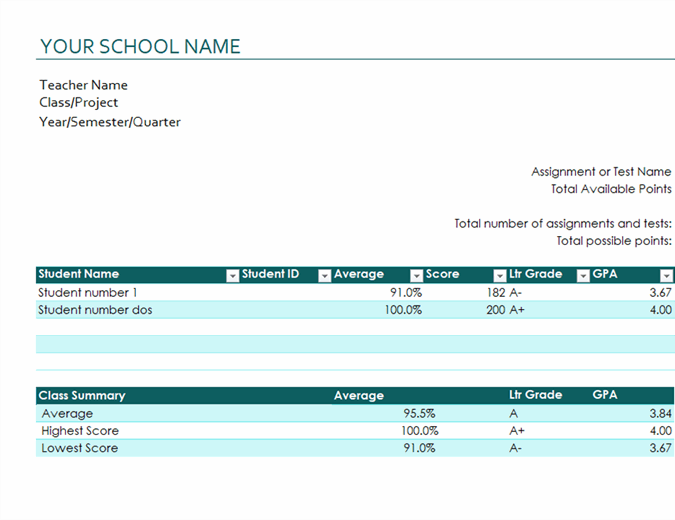Simplify the complex with data types in Excel. DOWNLOAD THE TEMPLATES NOW.

# Teacher's grade book (based on points)

Teachers can track students' grades with this accessible grade book template in Excel. Just enter each assignment and the total available points for each, and as you enter each student's scores, the average, letter grade, and GPA will be calculated. This free grade book template saves valuable time by calculating student grades based on points.

Excel